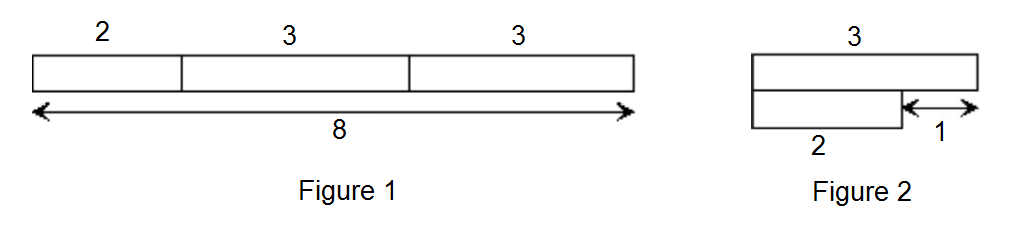# Efficient Measures

Probability Level 1Using one 2 meter length and two 3 meter length, I can measure 8 meters as shown in Figure 1. It is also possible to measure 1 meter, as shown in Figure 2. Which whole number length from 1 to 8 cannot be measured directly from one 2 meter length and two 3 meter length?

×